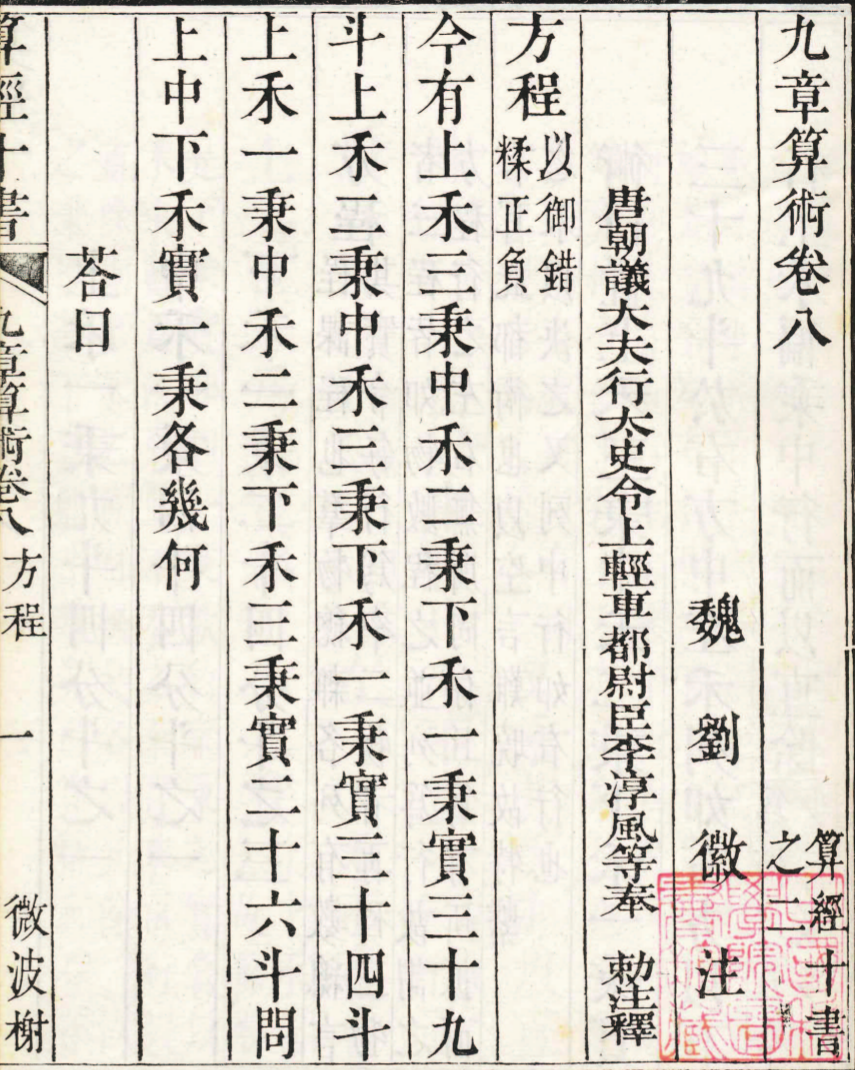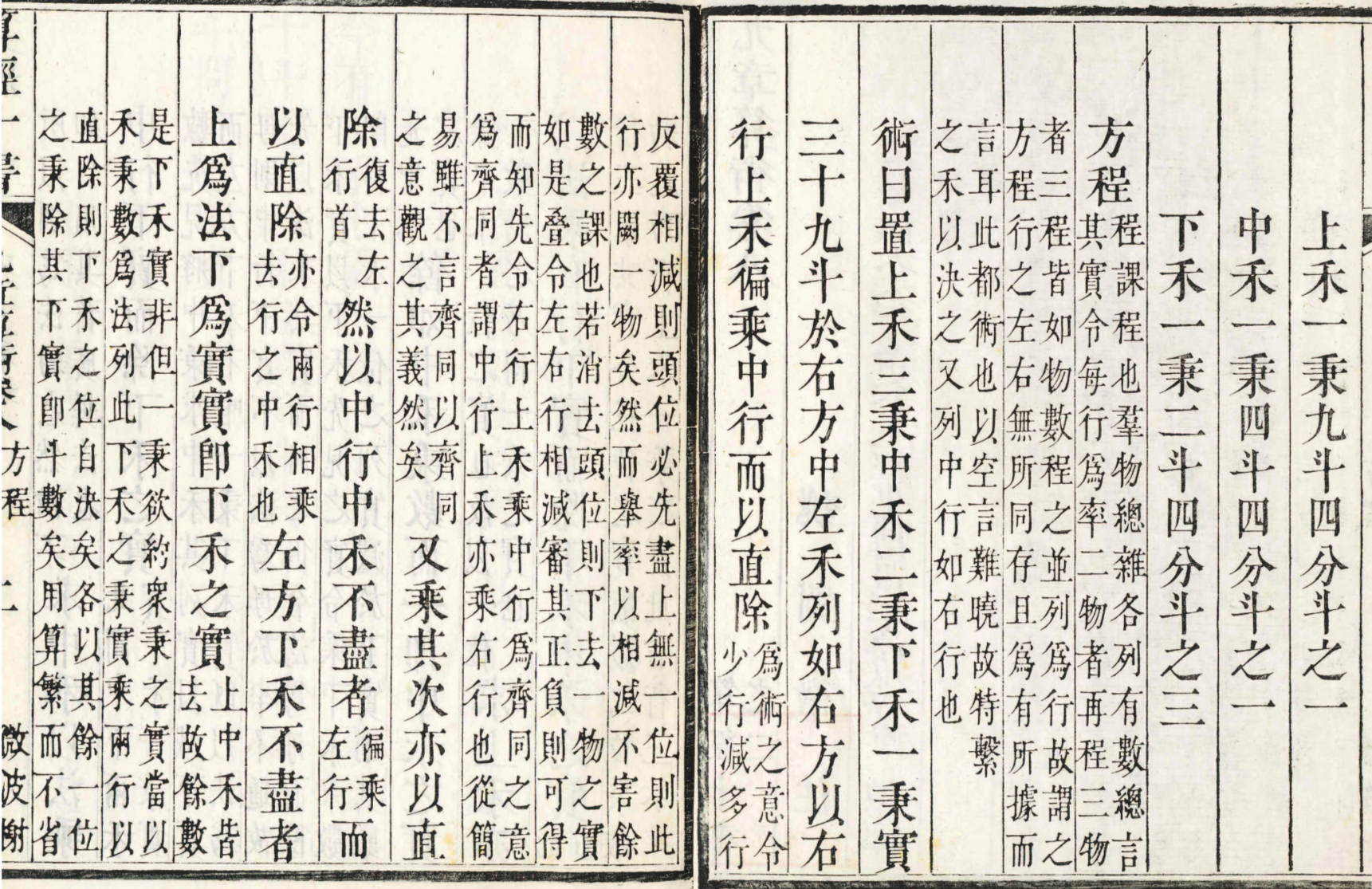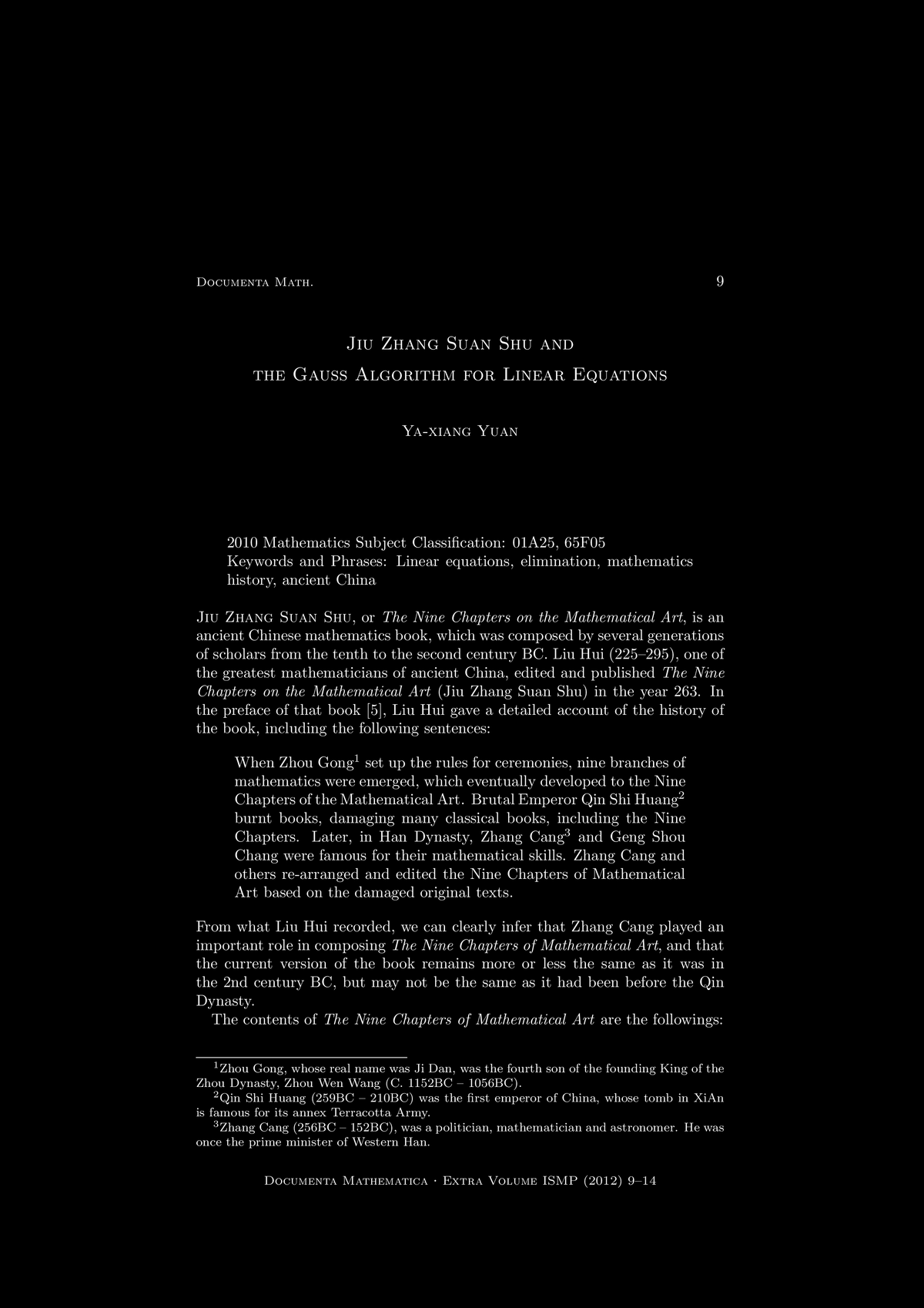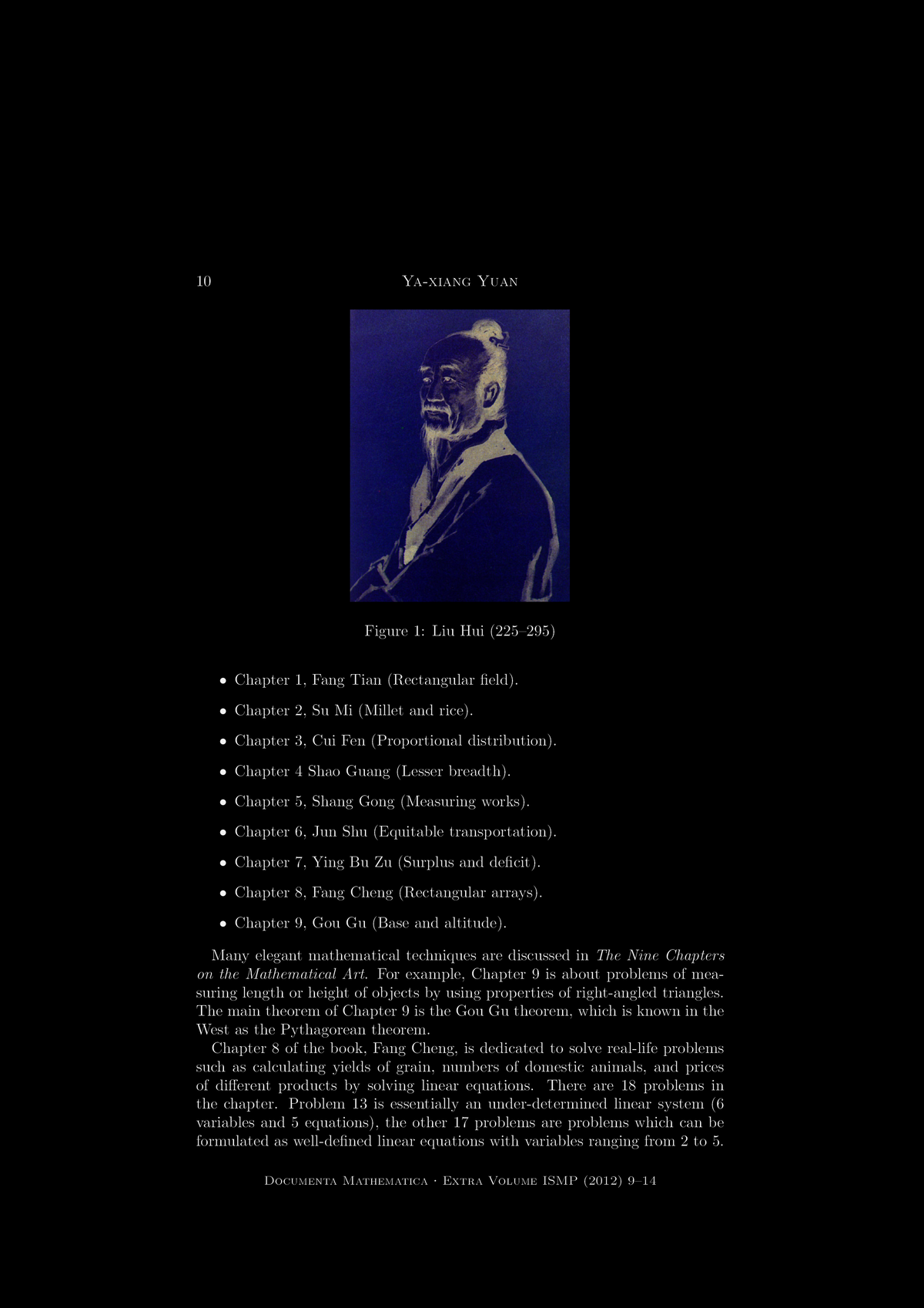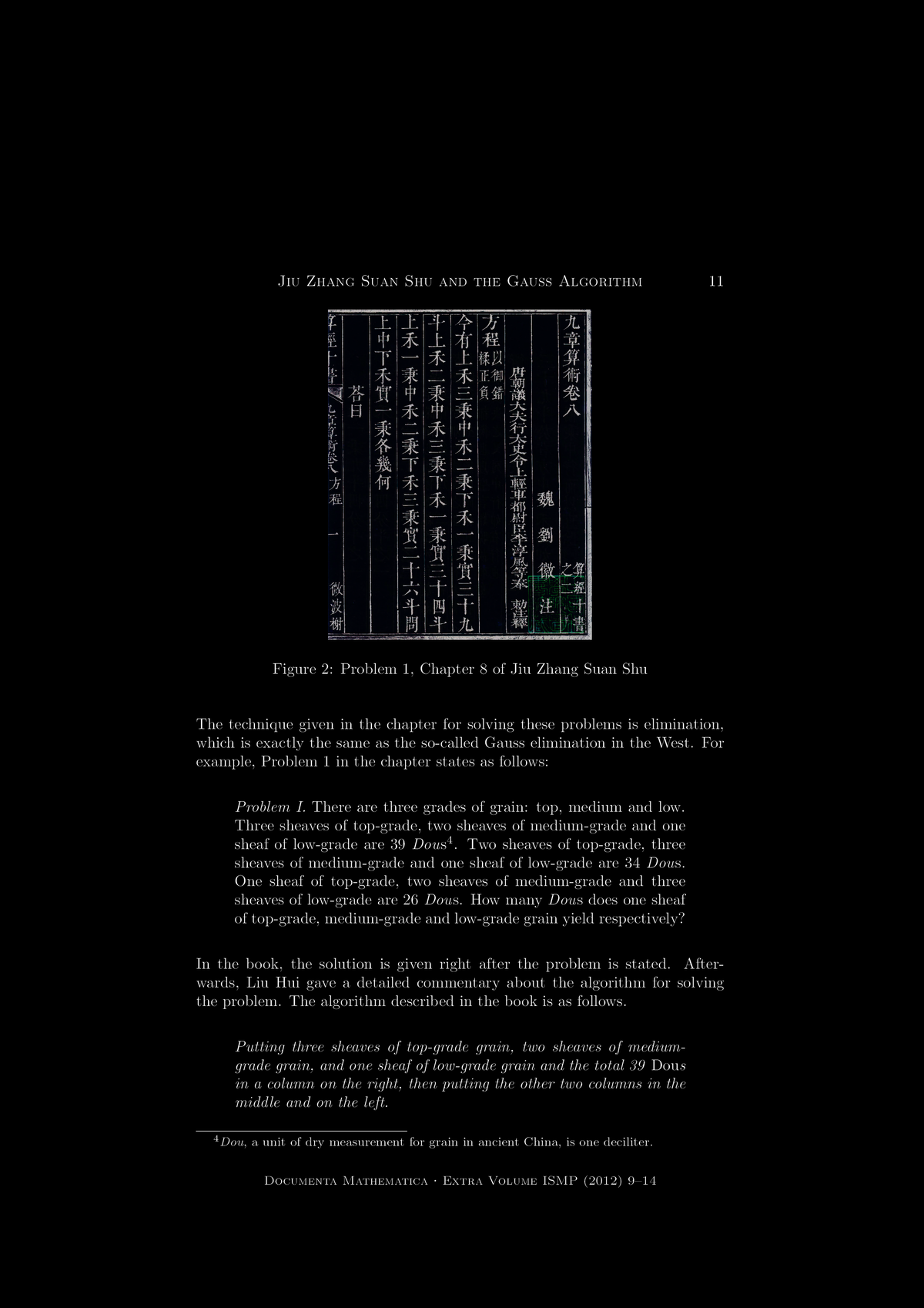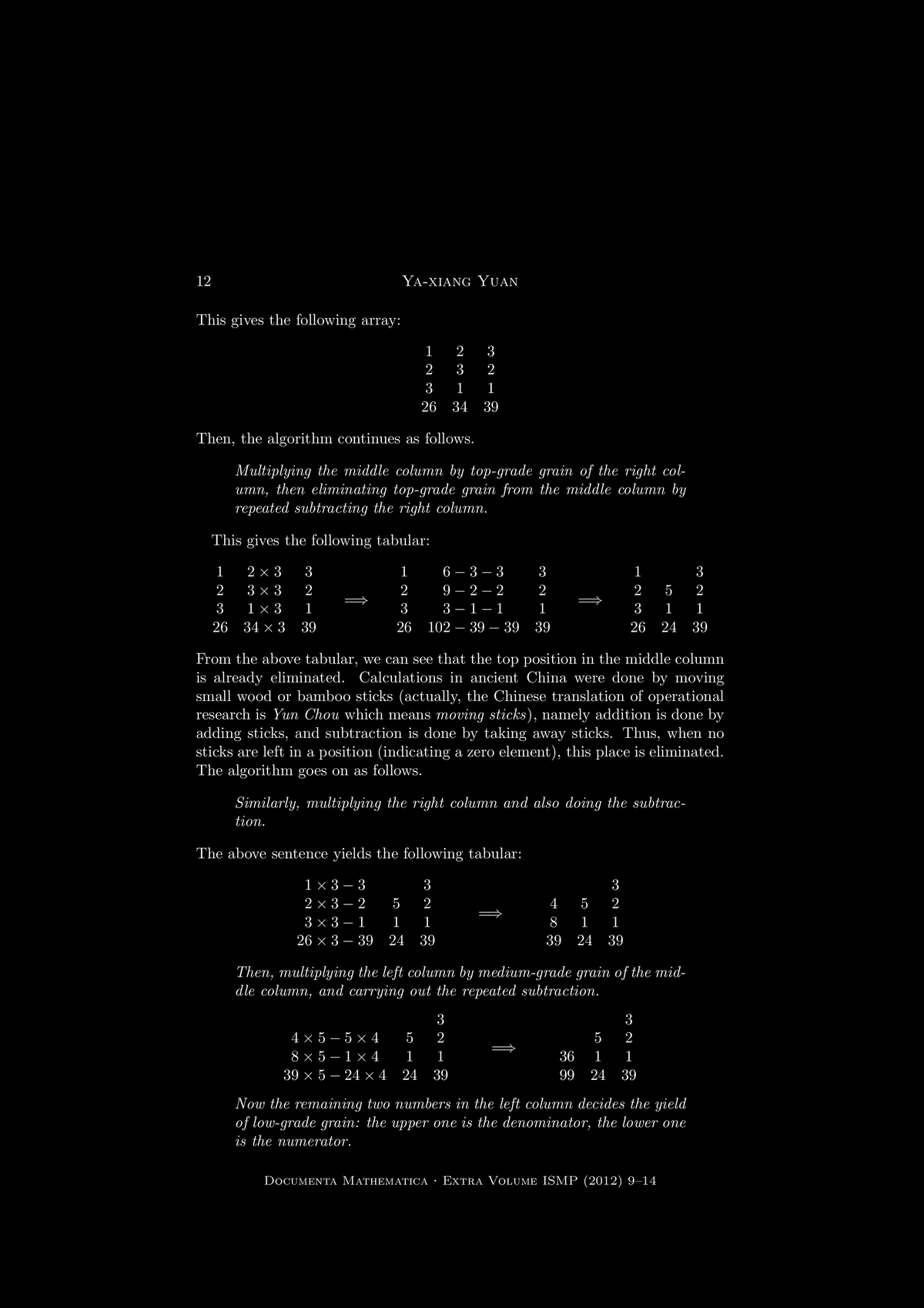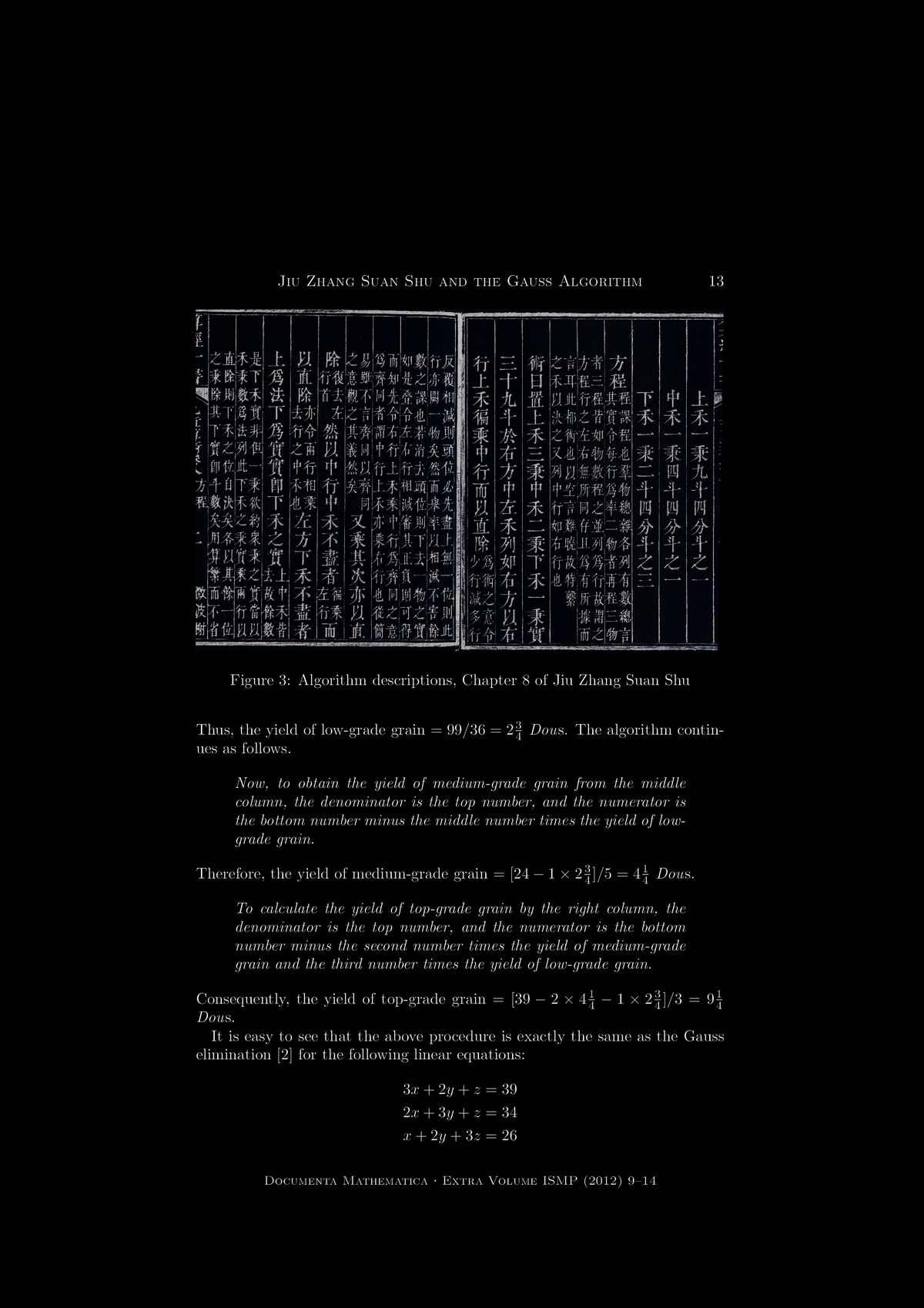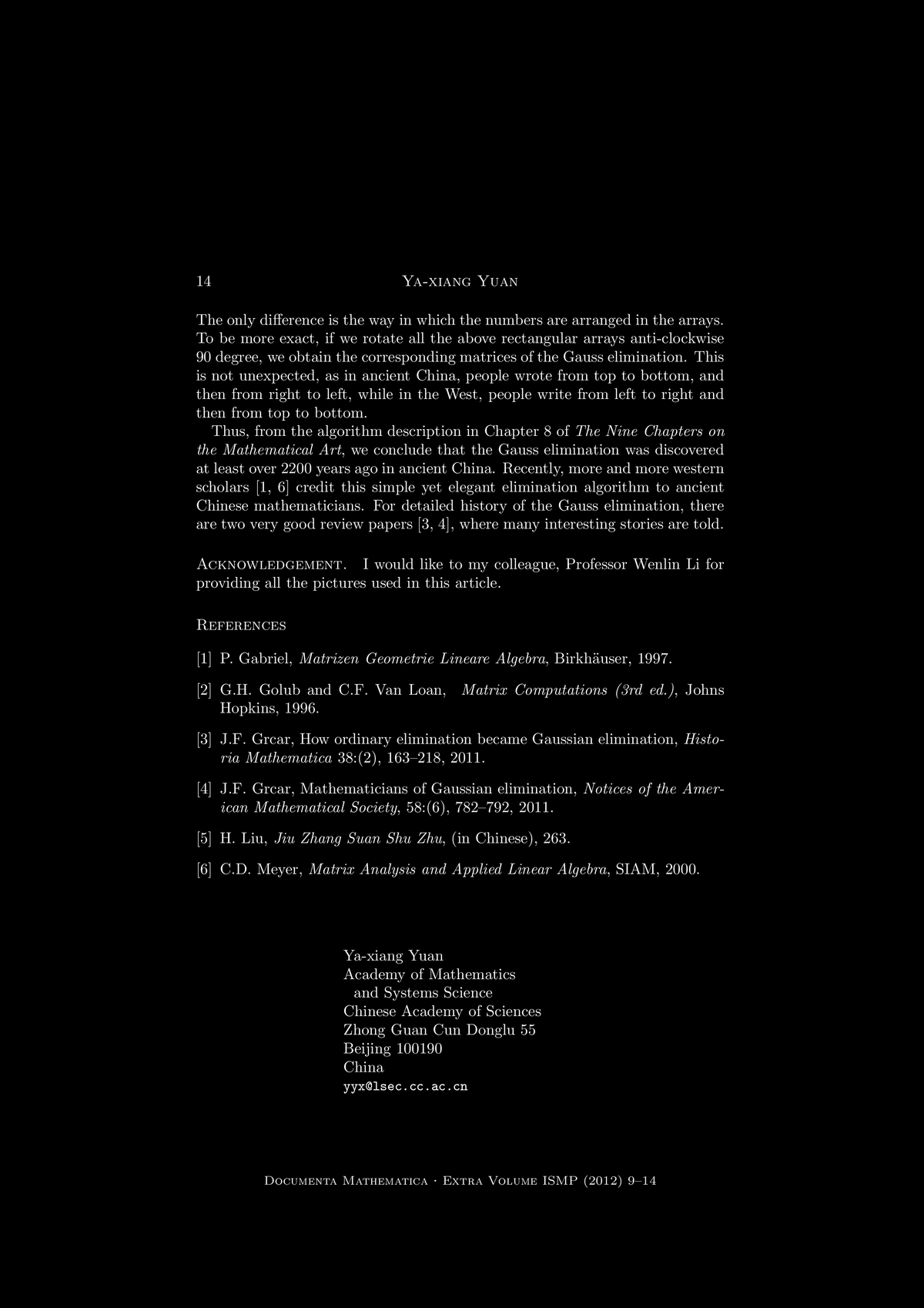M A T H 2 1 B
Mathematics Math21b Spring 2016
Linear Algebra and Differential Equations
Exhibit: 9 Chapters
Office: SciCtr 432
Here are larger versions of 3 figures given in the article "Jiu Zhang Suan Shu and the Gauss Algorithm for Linear Equations", by Ya-Xiang Yuan, Documenta Mathematica, Extra Volume ISMP (2012) 9-14.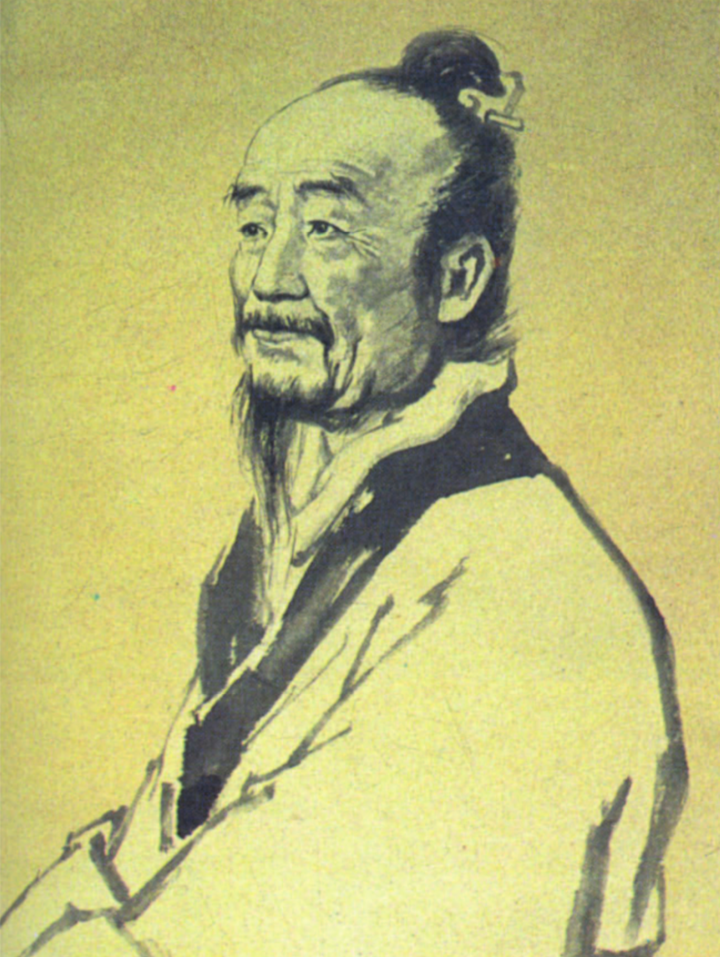Figure 1: Liu Hui 225-295, edited and published the Nine Chapters (Jiu Zhang Suan Shu) in 263. Here is the problem
```  3x+2y+z=39
2x+3y+z=34
x+2y+3z=26
```